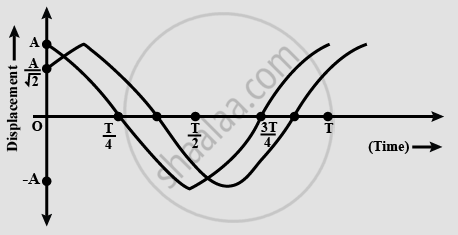# Two Particles Perform Linear Simple. Harmonic Motion Along the Same Path of Length 2a and Period T as Shown In the Graph Below. the Phase Difference Between Them is - Physics

MCQ

Two particles perform linear simple harmonic motion along the same path of length 2A and period T as shown in the graph below. The phase difference between them is ___________.#### Options

• pi/4 rad

• pi/2 rad

• (3pi)/4 rad

#### Solution

 pi/4 rad

Let the equation of waves be Asin( ωt + Φ )
For wave with peak at t = 0, the equation becomes,
y = Asin( ωt + 90° )
For the wave with displacement A/sqrt2 at  t = 0
A/sqrt2 = Asin(Φ)

⇒ Φ = 45° = pi/4
Hence this is the phase difference between the waves.

Concept: Differential Equation of Linear S.H.M.
Is there an error in this question or solution?